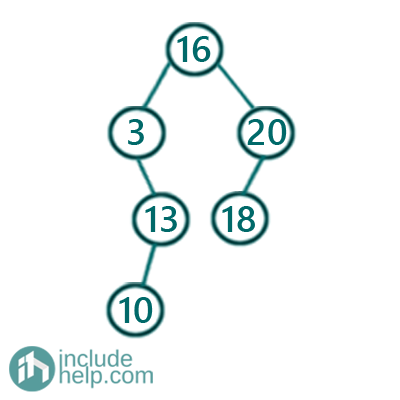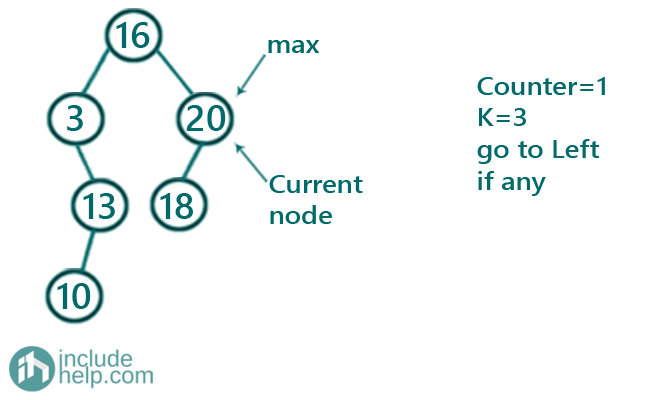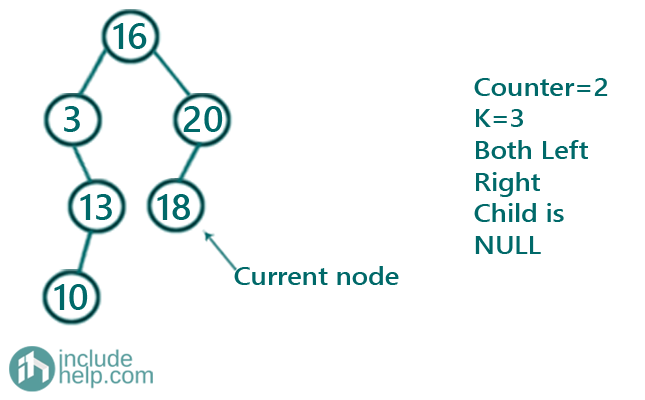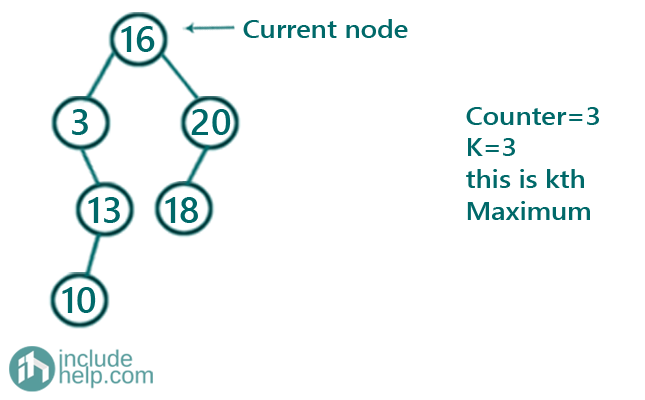# Kth Maximum in a Binary Search Tree

In this article, we are going to see how to find kth minimum in a Binary Search Tree?
Submitted by Radib Kar, on October 28, 2020

As we have discussed in our introductory article on Binary search tree, that finding kth maximum is an ADT operation. Here, we are going to see how we can find kth maximum in a given binary search tree.In the above binary search tree, the 3rd maximum is 16 and the 5th maximum is 10

So how can we find kth maximum given the binary search tree and the k as input?

The idea is reverse inorder traversal. If we do the reverse inorder traversal then we will reach to the maximum node in the BST first.  So, we need to keep a counter which will increment to find the kth maximum only. The reverse inorder traversal visits the BST nodes in reverse order(descending). So it will visit the 1st maximum, then the 2nd maximum and so on. So, our counter will determine the kth maximum. So the reverse order traversal is like below,

```void kthLargestRec(TreeNode* root,int K,int &count,int &ans){
//base case for reverse inorder traversal
if(!root)
return;
//reach the maximum node first doing reverse inorder traversal
kthLargestRec(root->right, K, count, ans);
//increment count
count++;
//Once the counter is K, we have reached our Kth maximum
if(count==K){
ans=root->val;
return;
}
kthLargestRec(root->left,K,count,ans);
}
```

Below is the visual illustration on how we find the kth maximum

Say k=3

And the given BST is the same as example

So, doing the reverse inorder traversal first we reach the maximum node. To show how-

First, we start from the root node

Then it goes to the immediate right child 20 and then the right child is NULL, so control comes back to node 20 and that's our first maximum. Counter is now 1Since the counter is not K, so it will recur for its left subtree

Now the current node is 18 and since there is no right subtree it's the node to be considered and the counter is now. So it's the second maximum.Now it will recur for the left subtree but since there is no left subtree the control will reach back to the root(16) as its right subtree is traversed already. So counter is 3 and that's why the root is our 3rd maximumIf we extend this to find 5th maximum, we can do a similar way and will find that 10 is our 5th maximum.

Below is the C+++ implementation of the above idea.

```#include <bits/stdc++.h>
using namespace std;

// tree node is defined
class TreeNode {
public:
int val;
TreeNode* left;
TreeNode* right;
TreeNode(int data)
{
val = data;
left = NULL;
right = NULL;
}
};

//reverse inorder traversal to find kth maximum element
void kthLargestRec(TreeNode* root, int K, int& count, int& ans)
{
if (!root)
return;
//reach the maximum node first doing reverse inorder traversal
kthLargestRec(root->right, K, count, ans);
//increment count
count++;
if (count == K) { //if it's K then the current node value is kth maximum
ans = root->val;
return;
}

kthLargestRec(root->left, K, count, ans);
}

// return the Kth largest element in the given BST rooted at 'root'
int kthLargest(TreeNode* root, int K)
{
int ans = INT_MAX;
int count = 0;
kthLargestRec(root, K, count, ans);

return ans;
}

int main()
{
TreeNode* root = new TreeNode(16);
root->left = new TreeNode(3);
root->left->right = new TreeNode(13);
root->left->right->left = new TreeNode(10);
root->right = new TreeNode(20);
root->right->left = new TreeNode(18);
int k = 3;
cout << "3rd maximum is: " << kthLargest(root, k) << endl;
k = 5;
cout << "5th maximum is: " << kthLargest(root, k) << endl;
return 0;
}
```

Output:

```3rd maximum is: 16
5th maximum is: 10
```